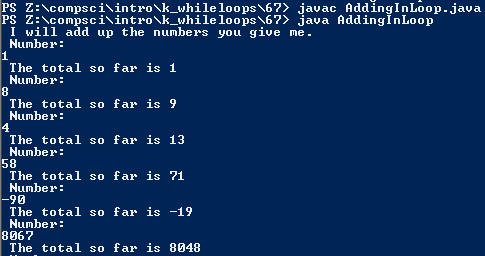# Assignemnt #67 and Adding in Loop

## Code

```      ///Name: Dakota Donahue
///Period: 5
///Date: 1/12/2016

import java.util.Scanner;

{

public static void main(String [] args)
{
Scanner keyboard = new Scanner(System.in);
int total = 0;

System.out.println( " I will add up the numbers you give me. ");
System.out.println( " Number: " );
int n = keyboard.nextInt();
total = n;
System.out.println( " The total so far is " + total );

while ( n != 0 )
{
System.out.println( " Number: " );
n = keyboard.nextInt();
total = ( n + total );
System.out.println( " The total so far is " + total );
}
}
}
```